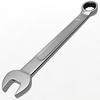28112.680

# Eibach® (11-14) Challenger V6 - 1.4" x 1.5" Pro-System-Plus Front/Rear Handling Lowering Kit

Regular price\$1,549.00
/
Shipping calculated at checkout.
• Question? Call us at (888) 257-1077 🏁
• Free Shipping USA (Exc. HI, AK, PR)

Compatible: (3.6L)

2011 - 2014 Dodge Challenger V6

STEP 1: MOTION RATIO

In developing a basic spring setup, you first step is determining your Motion Ratio. A different formula is needed for the type of suspension your race vehicle utilizes: A-arm or Beam axle. Please take into consideration the Angle Correction Factor in your computation.

### A-arm Suspension - (See Diagram 1)

MR
Motion Ratio
d1
Distance from spring centerlines to control arm inner pivot center (in) or (mm)
d2
Distance from outer ball joint to control arm inner pivot center (in) or (mm)### Beam Axle Suspension - (See Diagram 2)

MR
Motion Ratio
d3
Distence between spring centerlines (in) or (mm)
d4
Distance between tire centerlines### Angle Correction Factor

ACF
Angle Correction Factor
d3
Spring angle From Vertical (see diagram 1)
d4
Distance between tire centerlines#### DIAGRAM 1 (A-ARM SUSPENSION)

The motion ratio is a lever arm effect of the control arm acting on the spring. If the spring is mounted at an angle, the reduced motion of the spring must also be taken in account.#### DIAGRAM 2 (BEAM AXLE SUSPENSION)

The motion ratio of a live axle setup is shown here. Over two-wheel bumps, the motion ratio is 1:1. Over single wheel bumps and during body roll, the motion ratio as shown in step 1. The motion ratio is only used for calculating roll resistance, not for suspension frequencies.

## STEP 2: WHEEL RATE

Wheel Rate is the actual rate of a spring acting at the tire contact patch. This value is measured in lbs/inch or N/mm, just as spring rate is. The wheel rate can be determined by using the formula below.

### Wheel Rate (non beam)

WR
Wheel Rate (lbs/in) or (N/mm)
C
Spring Rate (lbs/in) or (N/mm)
MR
Motion Ratio
ACF
Angle Correction Factor## STEP 3: SUSPENSION FREQUENCY

Suspension Frequency refers to the number of oscillations or "cycles" of the suspension over a fixed time period when a load is applied to the vehicle

### Wheel Rate (non beam)

SF
Suspension Frequency (cpm)*
WR
Wheel Rate (lbs/in) or (N/mm)
Sprung Weight
Vehicle corner weight less unsprung weight### Tip 1: Calculation of Wheel Rate for a given frequency

WR
Wheel Rate (lbs/in) or (N/mm) (see step 2)
SF
SF Suspension Frequency (cpm) (see step 3)
Sprung Weight
Vehicle corner weight less unsprung weight### Tip 2: Calculation of Spring Rate needed for a given Wheel Rate

C
Spring Rate (lbs/in) or (N/mm)
WR
Wheel Rate (lbs/in) or (N/mm) (see step 2)
MR
Motion Ratio
ACF
Angle Correction Factor## Determining Spring Rate

All Eibach motorsport springs are tested between 20% and 70% of the spring’s total travel. This spring rate can be measured easily using the following steps:

Example Spring—Standard: 1200.250.0500 (12”Free Length, 2.5”ID, 500lb/in)

Metric: 0300.060.0100 (300mm Free Length, 60mm ID, 100N/mm)

### STEP 1: DETERMINE TRAVEL

For the spring to be rated, please refer to the specifications listed for your spring part number in this catalog and record the travel measurement.This number represents the total available travel from free height to coil bind. Our example spring travel measurements for standard: 6.25" metric: 146mm

### STEP 2: DETERMINE TEST RANGE

Calculate the first test point by taking 20% of 6.25" (which equals 1.25") or 20% of 146mm (which equals 29.20mm) and the second test point by taking 70% of 6.25" (which equals 4.375") or 70% of 146mm (which equals 102.20mm). The actual travel between these two points (3.125") or (73mm) is where we determine the spring rate.### STEP 3: SPRING RATE TEST

Preload the spring 1.25" or 29.20mm and record the force measurement. Continue to compress the spring an additional 3.125" (total compression of 4.375") or 73mm (total compression of 102.20mm) and record the force measurement. Calculate and record the difference in force between the two points (1.25">< 4.375") or (29.20mm>< 102.20mm). In our example the difference would be approximately 1565lbs or 7300N.

### STEP 4: SPRING RATE CALCULATION

With Eibach4s precise spring rate tolerance of +/- 2% (500 x 2% = 10 lbs) the spring rate should fall between 490 and 510 lbs/in (1565 / 3.125 = 500lb) or 95N and 105N (7300N / 73mm = 100N/mm).Install Guide:Warranty: